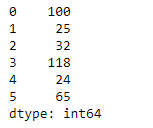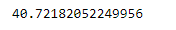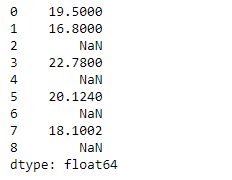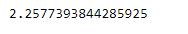Python | Pandas Series.std()

• Last Updated : 05 Feb, 2019

Pandas series is a One-dimensional ndarray with axis labels. The labels need not be unique but must be a hashable type. The object supports both integer- and label-based indexing and provides a host of methods for performing operations involving the index.

Pandas Series.std() function return sample standard deviation over requested axis. The standard deviation is normalized by N-1 by default. This can be changed using the ddof argument.

Syntax: Series.std(axis=None, skipna=None, level=None, ddof=1, numeric_only=None, **kwargs)

Parameter :
axis : {index (0)}
skipna : Exclude NA/null values. If an entire row/column is NA, the result will be NA
level : If the axis is a MultiIndex (hierarchical), count along a particular level, collapsing into a scalar
ddof : Delta Degrees of Freedom. The divisor used in calculations is N – ddof, where N represents the number of elements.
numeric_only : boolean, default None

Returns : std : scalar or Series (if level specified)

Example #1 : Use Series.std() function to find the standard deviation of the given Series object.

 # importing pandas as pdimport pandas as pd  # Creating the Seriessr = pd.Series([100, 25, 32, 118, 24, 65])  # Print the seriesprint(sr)

Output :Now we will use Series.std() function to find the standard deviation of the given Series object.

 # find standard-deviation along the# 0th indexsr.std()

Output :As we can see in the output, Series.std() function has successfully calculated the standard deviation the given Series object.

Example #2 : Use Series.std() function to find the standard deviation of the given Series object. We have some missing values in our series object, so skip those missing values.

 # importing pandas as pdimport pandas as pd  # Creating the Seriessr = pd.Series([19.5, 16.8, None, 22.78, None, 20.124, None, 18.1002, None])  # Print the seriesprint(sr)

Output :Now we will use Series.std() function to find the standard deviation of the given Series object.

 # find standard-deviation along the# 0th indexsr.std(skipna = True)

Output :As we can see in the output, Series.std() function has successfully calculated the standard deviation the given Series object. If we do not skip the missing values then the output will be NaN.

My Personal Notes arrow_drop_up